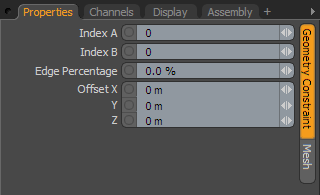# Geometry Constraints

Courtesy William Vaughan

#### Geometry Constraints Types

Vertex Position: This constraint attaches an item's center to a deformed vertex position of a Mesh Item.
Vertex Normal: This constraint matches an item's orientation to that of a specified vertex's normal direction. A vertex derives its normal direction as an average of all adjoining polygons.
Edge Position: This constraint attaches an item's center to a deformed edge of a Mesh Item.
Edge Normal: This constraint matches an item's orientation to that of a specified edge's normal direction. An edge derives its normal direction from an average of its two bordering polygons.
Polygon Position: This constraint attaches an item's center to the center of a deformed polygon of a Mesh Item.
Polygon Normal: This constraint matches an item's orientation to that of a specified polygon's normal direction.

#### Geometry ConstraintsIndex A: For vertices and polygons, the constraint uses this component index to get position and normal information. For edges, this is the index of the first vertex that defines the edge. By default, Modo uses the component index that is closest to the center of the item being constrained. To find the component index value for a particular component (vertex, edge, or polygon), make the appropriate selection of the item you wish to constrain to. The value shows in the info form within the Info and Statistics panel.

Index B: This index is used only for edges. It specifies the second vertex to use for the edge. This can be any vertex in the same mesh; the vertices do not need to be connected.

Edge Percentage: This is only used for Edge constraints. With this specified, the constraint can use an interpolated position (or normal) between the first and second vertices that define the edge. You can use this to slide the constrained item along the edge (for example, with 0% as the start of the edge and 100% as the end of the edge).

Offset: This is a world offset usually set when the constraint is applied with compensation on but can be used for further adjustment if required.